﻿ Solving Chemical Equilibria in Titrimetry with the Inclusion of Ionic Strength Using OCTAVE and EXCELPublications are Open
Access in this journal
Article Versions
Export Article
• Normal Style
• MLA Style
• APA Style
• Chicago Style
Open Access Peer-reviewed

### Solving Chemical Equilibria in Titrimetry with the Inclusion of Ionic Strength Using OCTAVE and EXCEL

Juan José Baeza-Baeza , Olivia Pini, Francisco F. Pérez-Pla, María Celia García-Álvarez-Coque
World Journal of Chemical Education. 2021, 9(3), 81-87. DOI: 10.12691/wjce-9-3-3
Received September 10, 2021; Revised October 14, 2021; Accepted October 24, 2021

### Abstract

Although Volumetric Analysis is one of the most widely used analytical techniques, there is limited published work on the use of computer software for simulation. In the few reports found in the literature, simplifications are often made for data treatment, due to the complexity of the equilibria involved. This work reports the application of a previously published methodology for calculating the concentration of chemical species, in multi-equilibrium problems, to simulate titration curves which describe the successive changes experienced in the solutions that are titrated. Two examples are solved with the help of the fsolve function in the open source computer programming language OCTAVE, and with the Solver tool in the EXCEL spreadsheet. Given that both the proposed methodology and the two computational platforms are capable of addressing complex systems without the need for simplifications, three important factors related with titrations can be considered: the behavior of the chemical indicator, the concurrence of collateral equilibria that can significantly affect the main titration reaction, and the effect of the ionic strength to explain deviations from ideality.

### 1. Introduction

The calculation of the concentrations of chemical species at equilibrium is of great interest in General Chemistry and several other scientific fields 1, 2, as Analytical Chemistry, Environmental Sciences, Chemical Engineering and Biochemistry, to study very different aspects, such as the interaction of proteins 3, preparation of buffers 4, determination of equilibrium constants 5, effect of pollutants in the environment 6, and supramolecular Chemistry 7. It is, therefore, a basic skill in the training of Chemistry students. Volumetric Analysis, also known as Titrimetry, is an area of Analytical Chemistry that relies on chemical equilibria. In this technique, an analyte is quantified by the volume of a standard solution required to react with it. Although it is one of the oldest methods, Titrimetry is often used because of its speed and simplicity, with little loss in accuracy and precision.

The computation of concentrations in equilibrium can be challenging for students, due to its dynamic nature and the need for expert handling of algebraic concepts 8, 9, 10. Therefore, owing to its complexity, the treatment of chemical equilibria is usually simplified in order to reduce the degree of numerical calculation. This makes them accessible to students, who are often struggling to solve conceptual chemical issues, for which higher-order thinking and cognitive skills are needed. In Titrimetry, the difficulty of mathematical treatments increases, since they must be applied to successive additions of titrants, and ideally, they must take into account the color changes of the indicator around the equivalence point. On the other hand, a significant frequent simplification is the ideality assumption that allows concentrations and activities to be equal, which can cause inaccuracies when intermolecular interactions are significant.

With increasingly available and easy-to-use computer software, these problems can be avoided. The programs presented in this work allow calculations for both simple and complex equilibria, regardless of the mathematical background of the students, focusing on the chemical equilibria involved. Both the methodology and the computational platforms make it possible to treat complex chemical systems, without the need for simplifications and generalizations. Since no simplifications should be made, emphasis will be placed on the behavior of the indicator, the concurrence of collateral equilibria, and the effect of the ionic strength to explain deviations from ideality. The study of these important factors do not appear prominently in the literature, even if their inclusion allows a more thorough and complete analysis of each chemical problem.

In this work, an open source computer language (OCTAVE) is applied together with the Solver tool in the EXCEL spreadsheet, to solve Volumetric Analysis problems, which may be of interest within an Analytical Chemistry course. The approach complements a previous report that did not consider the ionic strength 11. Now a more rigorous treatment can be carried out, which allows the study of equilibrium systems regardless of the number and type of reactions involved. On the other hand, the teaching of Titrimetry can be facilitated, since the treatment is unified whatever the type of equilibria involved. It also allows the study of all factors that influence a titrimetric procedure, such as the values of the equilibrium constants, the concentration of the reagents and the properties of the indicator. The described procedure is general and can be applied to any chemical equilibrium system if the reactions involved and their equilibrium constants are known. The two examples shown in this work correspond to acid-base and precipitation titrations, with quite different indication procedures.

### 2. Theory

Equilibrium problems can be addressed by solving a system of non-linear algebraic equations, consisting of the expressions for the equilibrium constants and various balances. One possibility is to use the extent of reaction balances 12 to obtain an equation associated to each chemical species. However, this gives rise to a complex system of equations, with many unknowns of different nature (concentrations and extent of reaction at equilibrium). A more convenient option is expressing the conservative mass balances, and charge, proton or electron balances, along with stoichiometric relationships between the species involved 13. These balances are just linear combinations of extent of reaction balances 14. This approach results in a system with as many equations as there are species to be determined.

In this work, the effect of the ionic strength is included without the need to increase the number of variables. This improves the method developed previously, where the ionic strength was taken as a variable 15, which has been applied for both teaching and research purposes 4, 16, 17. The resolution of the system of non-linear equations can be done with the Solver tool of the EXCEL spreadsheet, or by applying the OCTAVE fsolve function 11 or other similar software for technical calculation.

2.1. Taking into Account the Ionic Strength

In chemical equilibria, due to ionic interactions, activities (ai) should be used instead of concentrations ([Ai]). Both are related through the activity coefficient (fi), as follows 18:(1)

Several equations have been suggested to evaluate the activity coefficients 5, 18, 19, 20. In this work, the Davies equation 19 is used:(2)

where zi is the ionic charge, and I the ionic strength defined as:(3)

N being the number of charged species. Another simple equation is based on the so-called Bates-Guggenheim convention 20:(4)

More complex equations or algorithms can also be used 5, 18, to deal with solutions at high ionic strength. However, Eqs. (2) and (4) have shown good results in predicting the pH of acid-base buffers 15.

The concentrations are transformed into activities according to Eq. (1). In logarithmic form: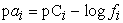(5)

where(6)

In this way, as the ionic strength is a function of the ion concentration, and the activity is a function of the ionic strength, pC can be taken as the final variable to be determined.

2.2. Procedure for Solving Equilibrium Problems

The approach described in this work follows a systematic step-by-step procedure, similar to the approach reported in Ref. 11, but now the activity coefficients are used. An essential step in the procedure is the implementation of the normalized null balanced equation system. This is necessary because of the large scale difference between the concentrations and the equilibrium constants. The reliability of the results is also increased, since all balances have the same weight in the sum that is minimized. It also allows the inclusion of all species in the optimization process, which makes the inclusion of the ionic strength as variable, as is done in Ref. 15, unnecessary. To obtain reliable results, initial seed concentrations close to the actual values should be entered. When a titration curve is obtained, the process is applied for each added volume of titrant, using the dilution factors to account for the increase in volume throughout the titration. Obviously, both computational platforms (OCTAVE and EXCEL Solver) should produce identical results, although they are quite different. Excel is a popular spreadsheet that allows direct computation of each point in the titration curve, one by one. OCTAVE is an open source language with great flexibility that allows the continuous computation of all titration points in a range of volumes. It is more complex than the EXCEL Solver, since it requires knowledge of the programming language.

To improve the convergence and reliability of the results, all the parameters in the equilibrium expressions were used in logarithmic terms, as indicated in Eq. (5).

### 3. Results and Discussion

In this section, two examples are given to illustrate the proposed approach when applied to Volumetric Analysis, using the OCTAVE language and the Excel spreadsheet: (i) the volumetric determination of a monoprotic acid with a strong base, considering the effect on the equilibria of a visual indicator at low concentration, in order to study how it behaves throughout the titration, and (ii) the Volhard method that quantifies the silver ion by precipitation with thiocyanate as a titrant and ferric ion as a visual indicator added at a fairly large concentration, which affects the perception of the end point.

3.1. Titration of a Monoprotic Acid Using a Strong Base as Titrant and a Visual Indicator

First, a relatively simple problem will be addressed, consisting of a mixture of two weak monoprotic acids (HA, which is the analyte, and HIn, the added indicator) and a strong base (NaOH), which is the titrant. The behavior of the indicator system is an important factor in Titrimetry. Although the effect of the indicator on the titration equilibria is negligible if its concentration is small enough, the inclusion of the indicator in the system of equations will allow its effect being studied. The step-by-step procedure is as folows:

1. Obtain the initial concentrations: CIHA = 0.04 M, CIHIn = 10‒5 M, and CIOH = 0.05 M, with Vo = 25 mL, where the strong electrolyte NaOH is considered to be completely dissociated. All these concentrations will be reduced throughout the titration, according to the dilution factor:(7)(8)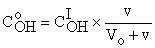(9)

and [Na+] = CoOH.

2. Write the R equilibria, and the corresponding expressions for the equilibrium constants. For the example, R = 3:(10)(11)(12)

3. Identify all species in equilibrium, S. The number of balances necessary to obtain the same number of equations as variables will be B = S – R. The species at equilibrium are S = 6: H+, OH, A, HA, In and HIn. This means that B = S – R = 6 – 3 = 3 balances are needed to solve the problem.

4. Set the initial values in pC units. As the initial solution is acidic, we propose pH = 3, pOH = 11, pA = 7, pHA = 2, pIn = 8, and pHIn = 5.

5. Establish the needed balances. As B = 3, use the mass balances for the analyte and indicator, and the charge balance:(13)(14)(15)

6. Calculate the ionic strength and the activity coefficients. The ionic strength expression will be here: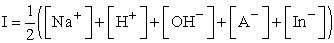(16)

The ionic strength is calculated from the initial value of pC for the six species in equilibrium. With this value, the activity coefficients can be evaluated from Eq. (2).

7. Calculate the activities of the ionic species. With the concentrations and the activity coefficients, the activities can be calculated in logarithm form for each species by applying Eq. (5).

8. Establish the system of normalized equations with the equilibrium constant expressed in logarithmic form, and the balances. Here, from Eqs (10), (11), (12) for the constants, and Eqs (13), (14) and (15) for the balances, the final system normalized to unity is: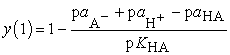(17)(18)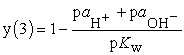(19)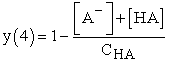(20)(21)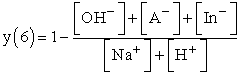(22)

9. Write the Excel spreadsheet or the OCTAVE files for the fsolve function (see Figure 1 and Table 1 for this example).

10. Run the Solver tool in Excel or the main file in OCTAVE to obtain the equilibrium concentrations.

In Titrimetry, the behavior of the indicator is of vital importance. To visualize the evolution of the acid-base indicator throughout the titration, it is convenient to represent the changes in the concentration of the acidic and basic colored species, superimposed with the titration curve. For this, the molar fractions of both colored species will be used: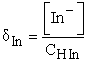(23)(24)

However, to facilitate observing the pH range of the indicator’s transition, the distribution diagram should be moved to the pH region where the indicator’s color changes. For this purpose, the interval of the molar fraction was matched with the transition interval of the indicator (pHb ‒ pHa), with pHb and pHa being the basic (upper) and acid (lower) limits of the color change, respectively: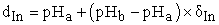(25)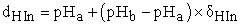(26)

Figure 1 shows the application of the proposed methodology using the Excel spreadsheet.

All the dynamics of the titration can be managed automatically through the OCTAVE loop commands by changing the initial concentrations, taking into account the dilution factor at each point of the titration. Equilibrium concentration values can thus be obtained for various volumes, and the corresponding titration curves are easily represented using the plot command. Table 1 shows the files for the OCTAVE code.

Figure 2 shows the changes in the concentrations of the chemical species (belonging to the analyte or indicator systems) with the pH throughout the titration. In the figure, the species in the upper line is the dominant (the one with the largest molar fraction). The mole fractions for both acid-base species are the same when their lines cross. The smaller the slopes of the lines near the crossing, the more progressive the indicator color change, which translates into a larger volume range during the transition. Therefore, cresol red (CR) undergoes an instantaneous change, while the change for bromocresol purple (BP) is gradual.

• Figure 1. Computation of the variables for a point of the titration curve (v = 20.1 mL), using the EXCEL spreadsheet. The orange arrows show the way the calculations are being performed (see the step-by-step procedure for solving equilibrium problems above)
• Figure 2. Titration curve for acetic acid (blue) and indicator transition calculated using the OCTAVE plot command: cresol red (7.0‒8.8, left), and bromocresol purple (5.2‒6.8, right). The pH at the equivalence point is 8.49. The modified molar fractions are represented for the acidic (yellow), and basic indicator species (red for cresol red and purple for bromocresol purple), according to Eqs. (25) and (26). Note that the molar fraction for the basic species varies between 0 and 1, and for the acidic species, between 1 and 0

It can be seen how CR has a sharp turn at the equivalence point (pH = 8.49), while BP begins its turn before the equivalence point, and progresses through a wide range of volumes. Note that both indicators have an end point close to the equivalence point: 20.005 mL for CR (Er% = 0.01%) and 19.94 mL for BP (Er% = ‒0.3%). However, the color change starts at 19.935 mL with CR and 16.0 mL with BP. Therefore, BP would lead to a long shift (colors mixed over a wide range), making it difficult to determine the actual end point.

3.1. Precipitation Titration: Determination of Silver Ion by the Volhard Method

The Volhard method is used as a back titration for the quantification of halide ions in a sample 21. Next, the proposed approach is applied to the calculation of the concentration of the chemical species involved in the titration of the silver ion in 25 mL of a solution containing 0.08 M AgNO3, 0.1 M NH4Fe(SO4)2 and 0.6 M HNO3, with a solution of 0.1 M KSCN. The equilibria involved (R = 9), for a complete description of the titration, are given in Table 2.

Since there are 14 variables and 9 equilibria involved, B = S – R = 14 – 9 = 5 balances are needed:(27)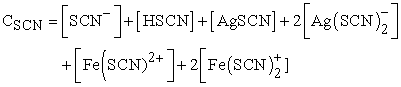(28)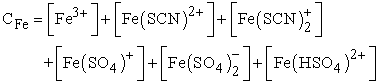(29)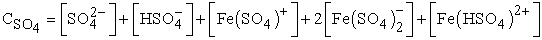(30)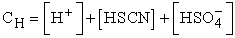(31)

The effect of the autoionization of water has not been taken into account, due to the high concentration of nitric acid. Therefore, the charge balance was not required. Null balances and OCTAVE scripts are provided in the Supporting Material. For this example, the molar fractions do not properly describe the indicator transition at the end point, due to the slow variation. To represent this transition, we propose instead, together with the titration curve, to draw a function that depends on the concentration of the red FeSCN2+ complex (used as indicator), as follows:(32)

Eq. (32) indicates the detectability of the complex (D), which depends on the molar absorbance of the red complex (1000 being an approximate value of the molar absorptivity) 5. The D value has been moved to match the equivalence point (pAg = 6).

Titration of the silver ion with thiocyanate, using Fe(III) as an indicator, is successful as the concentration of the red complex begins to increase at the equivalence point. Therefore, this can be accurately detected by the color change caused by complexation of the ferric ion with thiocyanate. In this example, the observable complex concentration (10‒5 M) is reached at 0.004 mL after the equivalence point, with an error of 0.02%. However, as seen in the figure, the red complex continues to increase its concentration, and therefore, the intensity of the color of the solution. For this reason, it is very important to use a silver-free reference solution to indicate the intensity of the color at which the titration should stop. When the initial Fe(III) concentration (i.e., the indicator) is lowered 100-fold, the final volume increases very little to exceed 0.05 mL (0.25% error).

• Figure 3. Titration of silver nitrate with potassium thiocyanate (blue), using ferric ion as indicator to form [Fe(SCN)2+] (red)

### 4. Conclusions

OCTAVE open source software allows a rigorous treatment of any equilibrium problem, without the need for deep mathematical knowledge. It takes only a simple algebra and basic rules for operating with logarithms to construct and manipulate the system of non-linear equations formed by the equilibrium constants, and charge and mass balances. Now students can give their full attention to understanding the chemical problem, and spend all their time discovering the involved species, equilibrium reactions, expressions of equilibrium constants, and conservation balances, which requires a correct understanding of the problem. Furthermore, the dynamic nature of equilibria can be studied by changing the initial conditions and calculating their effect assisted by graphics. It should be noted that there is no restriction on the type of reactions that can be included, only knowledge of the equilibrium constants is required. Students only need to check whether each included reaction is still valid under the final equilibrium conditions. For example, when an ionic solid is fully dissolved, its solubility product must be removed.

On the other hand, OCTAVE requires the student to build its own program, and the code to explicitly write the equations that govern the chemical system, instead of using software with hidden code that only allows changing the initial experimental conditions, without the need to know the real nature of the equilibrium problem. Students will also gain experience with a method that needs to solve a system of simultaneous equations using OCTAVE.

In this work, a complete treatment of the equilibria has been carried out, considering the ionic strength and the concurrence of collateral reactions. However, in many problems these effects can be ignored, as is the case of an acid-base titration involving low concentrations as in the shown example, where the ionic strength is below 0.05 M. This gives a relative error below 1.7% for the calculation of pH throughout the titration. On the other hand, in the Volhard example, an ionic strength of 0.9 M is reached, and the relative error in pC for the species with the highest charge (Fe3+) can exceed 40%.

### Supporting Information

The Supporting Information is:

1. Description of the Volhard method (DOCX)

2. OCTAVE and Excel files (ZIP)

### Statement of Competing Interests

The authors have no competing interests.

### Acknowledgements

This work was supported by Project PID2019-106708GB-I00, Ministry of Science and Innovation of Spain (State Research Agency: Ref. PROYECTO/AEI/ 10.13039/501100011033). Olivia Pini from the Imperial College London worked in the research as an Erasmus student to get her MSci in Chemistry.

### References

  Harris, D.C. and Lucy C.A., Quantitative Chemical Analysis, 10th ed, Macmillan Learning, New York, 2020. In article  Soustelle, M. Chemical Equilibria, in Chemical Engineering Series, Vol. 4, ISTE Ltd, London, 2015. In article View Article  PubMed  Ravera E., Carlon, A. and Parigi, G., “Pairwise binding competition Experiments for sorting hub-protein/effector interaction hierarchy and simultaneous equilibria”, J. Biomol. NMR 60, 29-36, 2014. In article View Article  PubMed  Ganesh, K., Soumen, R., Ravichandran, Y. and Janarthanan. “Dynamic approach to predict pH profiles of biologically relevant buffers”, Biochem. Biophys. Rep. 9, 121-127, 2017. In article View Article  PubMed  de Berg, K., Maeder, M. and Clifford, S., “The thermodynamic formation constants for iron(III) thiocyanate complexes at zero ionic strength”, Inorganica Chim. Acta 466, 249-253, 2017. In article View Article  Huysman, S., Vanryckeghem, F., De Paepe, E., Smedes, F., Haughey, S.A., Elliott, C.T., Demeestere, K. and Vanhaecke, L., “Hydrophilic divinylbenzene for equilibrium sorption of emerging organic contaminants in aquatic matrices”, Environ. Sci. Technol. 53, 10803-10812, 2019. In article View Article  PubMed  Todd, E.M. and Zimmerman, S.C., “Modeling the equilibria of complex supramolecular systems”, J. Chem. Educ. 86, 638-643, 2009. In article View Article  Özmen, H., “Determination of students’ alternative conceptions about chemical equilibrium: a review of research and the case of Turkey”, Chem. Educ. Res. Pract. 9, 225-233, 2008. In article View Article  Kousathana, M. and Tsaparlis, G., “Students' errors in solving numerical chemical-equilibrium problems”, Chem. Edu. Res. Pract. 3, 5-17, 2002. In article View Article  Tyson, L., Treagust, D.F. and Bucat, R.B. “The complexity of teaching and learning chemical equilibrium”, J. Chem. Educ. 76 (4), 554-558, 1999. In article View Article  Baeza-Baeza, J.J., Pérez-Pla, F. and García-Alvarez-Coque, M.C., “Teaching chemical equilibria using open source software OCTAVE”, World J. Chem. Educ. 3, 127-133, 2015. In article  Paz-García, J.M., Johannesson, B., Ottosen, L.M., Ribeiro A.B. and Rodríguez-Maroto, J.M. “Computing multi-species chemical equilibrium with an algorithm based on the reaction extents”, Comput. Chem. Eng. 58, 135-143, 2013. In article View Article  Baeza-Baeza, J.J. and García-Alvarez-Coque, M.C., “Systematic approach to calculate the concentration of chemical species in multi-equilibrium problems”, J. Chem. Educ. 88, 169-173, 2011. In article View Article  Baeza-Baeza, J.J. and García-Alvarez-Coque, M.C., “Extent of reaction balances: a convenient tool to study chemical equilibria”, World J. Chem. Educ. 2, 54-58, 2014. In article  Baeza-Baeza, J.J. and García-Alvarez-Coque, M.C., “Systematic approach for calculating the concentrations of chemical species in multi-equilibrium problems: inclusion of the ionic strength effects”, J. Chem. Edu. 89, 900-904, 2012. In article View Article  Ring, T. and Kellum, J.A., “Modeling acid-base by minimizing charge-balance”, ACS Omega 4, 6521-6529, 2019. In article View Article  PubMed  Martín-Yerga, D., Pérez-Junquera, A., González-García, M.B., Hernández-Santos, D. and Fanjul-Bolado, P., “In situ spectroelectrochemical monitoring of dye bleaching after electrogeneration of chlorine-based species: application to chloride detection”, Anal. Chem. 90, 7442-7449, 2018. In article View Article  PubMed  De Paula, J., Atkins, P. and Keeler, J., Physical Chemistry, Oxford University Press, Oxford, 2017. In article  Davies, C.W., “The extent of dissociation of salts in water. Part VIII: an equation for the mean ionic activity coefficient of an electrolyte in water, and a revision of the dissociation constants of some sulphates”, J. Chem. Soc. 2093-2098, 1938. In article View Article  Bates, R.G. and Guggenheim, E.A. “Report on the standardization of pH and related terminology”, Pure Appl. Chem. 1, 163-168, 1960. In article View Article  Haouet, M.N., Altissimi, M.S., Framboas, M., and Galarini, R., “Validation of the Volhard Method for chloride determination in food”, Accred. Qual. Assur. 11, 23-28, 2006. In article View Article  Cave, G.C.B. and Hume D.N.A., “Study of thiocyanatoargentate(I) ions by a solubility method”. J. Am. Chem. Soc. 75, 2893-2897, 1953. In article View Article  Stipp, S.L., “Speciation in the Fe(II)-Fe(III)-SO4-H2O system at 25°C and low pH: sensitivity of an equilibrium model to uncertainties”, Environ. Sci. Technol. 24, 699-706, 1990. In article View Article

Published with license by Science and Education Publishing, Copyright © 2021 Juan José Baeza-Baeza, Olivia Pini, Francisco F. Pérez-Pla and María Celia García-Álvarez-CoqueThis work is licensed under a Creative Commons Attribution 4.0 International License. To view a copy of this license, visit http://creativecommons.org/licenses/by/4.0/